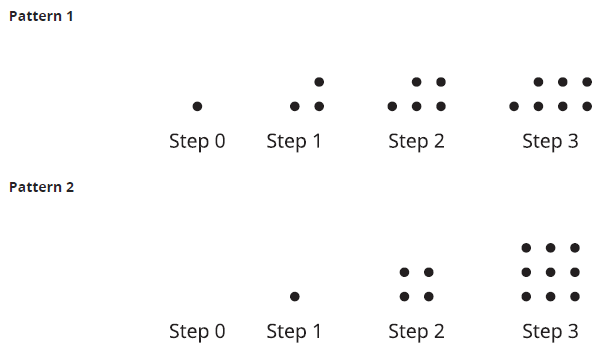# A.6.2.2 Patterns of Dots1. Study the 2 patterns of dots. a. How are the number of dots in each pattern changing? b. How would you find the number of dots in the 5th step in each pattern?

Complete the table with the number of dots in each pattern and the plot the relationship of step number and number of dots for pattern 1 and 2.

Explain why the graphs of the 2 patterns look the way they do.